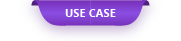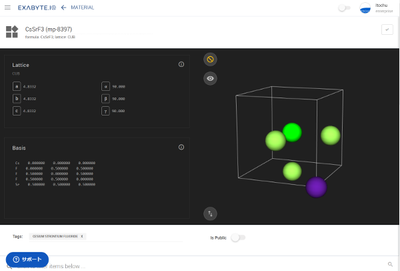Therefore, we verified whether it can be reproduced using Exabyte.io and Quantum ESPRESSO by calculation with pseudopotential.

# Comparison of WIEN2k and Quantum ESPRESSO of CsSrF3 with perovskite structure

#### Overview

K. Epharaim Babu et al. Calculate CsCrF3 of perovskite structure using WIEN2K at full potential . Therefore, we verified whether it can be reproduced using Exabyte.io and Quantum ESPRESSO by calculation with pseudopotential.

#### 1. Modeling

When creating a model, the crystal structure is created by importing the crystal structure from the Materials Project. You can search from the Materials Project database by simply entering "CsSrF3" in the Materials of the Exabyte.io item.

#### 2. Calculation

##### 2.1. Lattice constant optimization

To optimize the lattice constant of CsSrF3, use the work flow "Variable-call Relaxation". The pseudopotential used is the one that is the default setting in Exabyte.io.

Table 1 Comparison of lattice constants

Lattice constant [Å]
Experimental value4.83
WIEN2K 4.83
Exabyte.io & Quantum ESPRESSO4.82

Compare the calculation results. Even when the pseudopotential was used, the results were almost in agreement with the experimental values ​​and the results of the full potential.

##### 2.2. Bandgap

When finding the bandgap, use the work flow "Band Gap".

When calculating, you can use the "parent" function to calculate the bandgap based on the result of the job that optimized the lattice constant.

Table 2 Bandgap comparison

Direct band gap [eV]
WIEN2K 6.34
Exabyte.io & Quantum ESPRESSO6.26

Compare the calculation results. The results were close to the full potential results (Table 2).

##### 2.3. Band dispersion

When finding the band dispersion, use the work flow "Band Structure". The k-point path is also set automatically.